Journal of Measurements in Engineering

Published: 06 August 2021

# Traffic signal light detection and recognition based on canny operator

Liu Yuming1
Guo Shuqing2
1, 2Beihua University, Jilin, China
Corresponding Author:
Guo Shuqing
Views 524

#### Abstract

In this paper, five classical edge detection operators are compared, and then a traffic signal light detection and recognition scheme that can be used for intelligent connected vehicles is implemented. Firstly, the image to be processed is obtained by detecting the traffic signal light through the vision sensor. The image is preprocessed: the color space of the image is converted from RGB space to HSV space. Through the grayscale, histogram equalization, image binarization processing, using the morphological closure operation, the five operators are compared in noise sensitivity, positioning accuracy and signal-to-noise ratio, the Canny edge detection operator is selected for image edge detection, and the target recognition area is obtained. Finally, using the histogram drawn, the number of red, green and yellow pixel points in the histogram can be clearly counted, and the color with the largest number of pixel points can be identified as the color of the identified traffic signal light, and the identification of the traffic signal light can be completed. The actual pictures are simulated on the MATLAB, which verifies the feasibility of the proposed method of traffic signal light recognition method based on Canny operator in this paper, which can correctly identify the color of the traffic signal light.

## 1. Introduction

With the acceleration of urbanization and the widespread popularization of automobiles, traffic problem has become one of the most disturbing and serious problems in the world. At the same time, intelligent connected vehicles occupy an increasingly important position. Traffic signal light is one of the important information guidance of traffic signals. Accurate identification of traffic signal light in advance is conducive to the pre-planning of the path of intelligent networked vehicles, so as to decide the choice of vehicle parking or execution. Therefore, the study of traffic signal light identification technology also has important significance and good application prospects.

Many famous researchers at home and abroad have put forward the recognition algorithm of traffic signal lights. The existing traffic signal lamp detection methods mainly include color feature-based detection methods and shape feature-based detection methods. In 2006, Maphipa R. Yelal et al. realized the detection of traffic signal lights based on the method of color segmentation . Firstly, the algorithm converts RGB images into Lab images, performs color segmentation in the Lab color space, clustering the pixels and obtaining the candidate area of the signal lamp. Then, the edge information of the candidate area is analyzed, and finally the identification and detection of the traffic signal lamp is completed. This method reduces the parameters required for color segmentation and simplifies the debugging process by converting the color space, but the disadvantage is that the performance of the program cannot be completely guaranteed in the complex environment. Kuo-Hao Lu et al. also used color space to extract candidate areas of traffic signal lights . Compared with the previous method, they labeled the candidate areas and carried out template feature matching of circle and arrow shapes in each candidate area according to self-defined shape feature rules. This method can classify the circular and arrowhead shapes of traffic signal lights when the image of traffic signal lamp is large (the area of the signal lamp is > 165 pixels). However, the recognition distance should not be too far when collecting by on-board camera, which is not conducive to the path planning and decision-making of intelligent vehicles at intersections. In 2009, Masako Omachi proposed traffic signal light detection based on color and edge . The color was firstly normalized and then segmented to obtain candidate regions of the color features of traffic signal lights. Sobel algorithm is used to extract the edge of the candidate region, and then Hough transform is used to identify the circular region and complete the detection of traffic signal lights. The disadvantage of this algorithm is that the anti-interference is not strong and the interference of other external factors such as car lights cannot be eliminated. Raoul de Charette et al. analyzed that most of the traffic signal light identification methods studied by predecessors are based on suspended traffic signal lights . However, in the field of view of the on-board camera, the background of suspended signal lights is the sky with relatively simple background environment, which is not very suitable for the urban road environment with complex background. Therefore, a circular traffic light recognition algorithm based on brightness and shape is proposed. The algorithm first converts RGB images into grayscale images, then carries out top hat transformation on the grayscale images obtained, and then carries out binarization processing to obtain binarization images. Then, it filters the connected areas through morphological filtering, and finally uses the adaptive template to match ATM for recognition. The algorithm has strong robustness, but the method cannot recognize the traffic lights in the weather with too much illumination and in the environment with too much illumination.

Previous schemes proposed by researchers from all over the world have strong identification capability, but it is difficult to quickly and accurately identify them in some harsh environments or complex working conditions. To solve these problems, this paper to simplify the recognition process, make the recognition of traffic lights time shortened, and the improved accuracy under the condition of long distance, the scheme of this paper is proposed as follows: Traffic signal light detection is carried out through the vision sensor, and the collected images are grayscale, histogram equalization, binarization and morphological closing operation. By comparing five classical edge detection operators, Canny operator is selected for image edge detection, and then the method of extracting the number of pixels is used. Determine the color with the largest number of pixels to be the recognized color. The feasibility and accuracy of the scheme proposed in this paper are verified by simulation on the MATLAB simulation platform for multiple actual traffic signal light images taken at intersections, and the color recognition of traffic signal lights is completed.

## 2. Comparative analysis of five image edge detection operators

In the theory of digital image processing, image edge is one of the basic features of the image. Image edge detection is not only the detection of the boundary, but also the place where the attributes of two regions mutate. Image edge detection is an accurate and efficient detection of the boundary between a certain type of specific object and its background through scientific and reasonable algorithm. The main steps can be divided into image analysis, image recognition, boundary reconstruction and extraction. At present, digital image edge detection methods can be mainly divided into three categories: One is based on a fixed local operation method, which is mainly based on the local simplified mathematical basis and its principle, such as differential and integral method and fitting method. This kind of method belongs to the classical edge detection method. The second is global extraction method based on energy minimization rule, the basic idea to use rigorous mathematics theory method is reasonable and accurate analysis to the problem, its most optimal detection based on one dimensional cost function is given, from the point of view of the global optimal for image edge detection, this kind of method for mathematical logic is relatively tight, Such as neural network analysis and principal component analysis; The third kind of method is the new image edge detection method supported by the high-tech technologies that have developed rapidly in recent years, such as wavelet transform and mathematical morphology. This kind of algorithm can combine the traditional simple image edge detection theory with the latest theoretical methods, and then integrate into the latest developed deep learning technology. Finally, it can provide a reference for the basic method of image edge detection theory in artificial intelligence learning.

From the concept of image edge, we can know that image edge is the position where the gray level of the image changes significantly. The algorithm principle based on image edge feature and gradient theory is as follows:

1
$\nabla f\left(x,y\right)=\frac{\partial f}{\partial x}i+\frac{\partial f}{\partial y}j={f}_{x}\left(x,y\right)i+{f}_{y}\left(x,y\right)j.$

At the same time:

2
$E\left(x,y\right)=\sqrt{{f}_{x}^{2}\left(x,y\right)+{f}_{y}^{2}\left(x,y\right)}.$

It is the amplitude of the gradient $\nabla f\left(x,y\right)$, $E\left(x,y\right)$ can be used as an edge extraction operator. For the sake of memorization, we can simplify the calculation and define $E\left(x,y\right)$ as the sum of the absolute values of the two, namely:

3
$E\left(x,y\right)=|{f}_{x}\left(x,y\right)|+\left|{f}_{y}\left(x,y\right)\right|.$

According to the above theory, many image edge extraction algorithms are derived. As for the dynamic change of image gray level, it can be accurately and objectively expressed by the gradient of image gray level function. Therefore, the image edge extraction algorithm can be obtained by the image local differential technology, which is convenient, practical and widely used at present.

### 2.1. Canny edge detection operator

Canny operator is an image edge detection algorithm proposed by John Canny in 1986. At present, it has become one of the classic algorithms in the field of digital image processing. Canny edge detection operator is a multi-level detection operator. Its basic principle and basic processing flow are as follows: Gaussian smoothing filter is carried out on the original image to smooth the image and filter out noise; Then, the gradient intensity and direction of each pixel were calculated respectively. Then, the non-extreme suppression technology was used to eliminate the influence of stray edges, and the real and potential edges were determined by double threshold detection. Finally, edge detection was completed by suppressing isolated weak edges, and the Canny edge detection processing image was obtained .

1) Gaussian filter smoothing the image.

Canny operator uses Gaussian filter to reduce the impact of noise, through the convolution processing with the image, filter out the noise in the image, and smooth the image.

2) Calculate the gradient amplitude and direction of the image.

When the Canny operator obtains the gradient image, the 2×2 template is used to calculate the gradient amplitude and gradient direction of the gray image. The gradient value in both horizontal and vertical directions is:

4
$\begin{array}{l}{G}_{x}\left(i,j\right)=\left[I\left(i,j+1\right)-I\left(i,j\right)+I\left(i+1,j+1\right)-I\left(i+1,j\right)\right]/2,\\ {G}_{y}\left(i,j\right)=\left[I\left(i,j\right)-I\left(i+1,j\right)+I\left(i,j+1\right)-I\left(i+1,j+1\right)\right]/2.\end{array}$

At this time, the magnitude of the gradient at $\left(i,j\right)$ is $G\left(i,j\right)$, the gradient directions $\theta \left(i,j\right)$ are respectively:

5
$\begin{array}{l}G\left(i,j\right)=\sqrt{{G}_{x}^{2}\left(i,j\right)+{G}_{y}^{2}\left(i,j\right)},\\ \theta \left(i,j\right)=\mathrm{a}\mathrm{r}\mathrm{c}\mathrm{t}\mathrm{a}\mathrm{n}\left(\frac{{G}_{y}\left(i,j\right)}{{G}_{x}\left(i,j\right)}\right).\end{array}$

3) Non-maximum suppression of the gradient amplitude is performed.

A 3×3 template window is used for the small neighborhood in the gradient amplitude graph $G\left(i,j\right)$, in which the central pixel is compared with its adjacent pixels in the gradient direction. If the value of the center pixel is not greater than the value of the adjacent pixels along the gradient, it is set to zero. Otherwise, this is a local maximum, so leave it there.

4) The double threshold method detects and connects the edges.

After non-maximum suppression, the image is extracted by double threshold with high threshold $Th$ and low threshold $Tl$, and the false edge is eliminated and the discontinuous edge is connected. If the gradient value of the edge point after non-maximum suppression is greater than the high threshold, it is retained as the edge point. The point where the gradient value is less than the low threshold value is deleted; For a point whose gradient value is between two thresholds and is adjacent to the edge point, it is necessary to judge whether there is any edge pixel greater than the high threshold value in its 8 neighborhoods. If there is, it will be retained as the edge point, otherwise it will be deleted.

### 2.2. Kirsch edge detection operator

Kirsch operator is one of the classical edge detection operators, which is still widely used today. In 1971, R. Kirsch proposed a method of calculating the gradient of image pixels in clockwise cycle to carry out edge detection. Assume a 3×3 neighborhood of one pixel, and take the gradient size of the edge as:

6
$m\left(i,j\right)=\mathrm{m}\mathrm{a}\mathrm{x}\left\{1,\mathrm{m}\mathrm{a}\mathrm{x}\left\{\left|5{s}_{k}-3{t}_{k}\right|,k=0,1,\dots ,7\right\}\right\}.$

In the formula:

7

When $k=$ 0, 1,..., 7, the following eight templates are used:

8
$\begin{array}{l}\underset{{M}_{0}}{\left[\begin{array}{lll}5& 5& -3\\ 5& 0& -3\\ -3& -3& -3\end{array}\right]}\underset{{M}_{1}}{\left[\begin{array}{lll}5& 5& 5\\ -3& 0& -3\\ -3& -3& -3\end{array}\right]}\underset{{M}_{2}}{\left[\begin{array}{lll}-3& 5& 5\\ -3& 0& 5\\ -3& -3& -3\end{array}\right]}\underset{{M}_{3}}{\left[\begin{array}{lll}-3& -3& 5\\ -3& 0& 5\\ -3& -3& 5\end{array}\right]}\\ \underset{{M}_{4}}{\left[\begin{array}{lll}-3& -3& -3\\ -3& 0& 5\\ -3& 5& 5\end{array}\right]}\underset{{M}_{5}}{\left[\begin{array}{lll}-3& -3& -3\\ -3& 0& -3\\ 5& 5& 5\end{array}\right]}\underset{{M}_{6}}{\left[\begin{array}{lll}-3& -3& -3\\ 5& 0& -3\\ 5& 5& -3\end{array}\right]}\underset{{M}_{7}}{\left[\begin{array}{lll}5& -3& -3\\ 5& 0& -3\\ 5& -3& -3\end{array}\right].}\end{array}$

Multiply ${M}_{0}$-${M}_{7}$ by each of the corresponding elements in the neighborhood, the maximum value of the 8 calculated results is used as the approximate gradient value of the center pixel, and the corresponding direction is used as the edge direction. The eight convolution kernels form the Kirsch operator. During edge extraction, the 3×3 neighborhood corresponding to each pixel in the image is convolved with these 8 templates, and then the maximum value is output .

### 2.3. Sobel edge detection operator

Sobel edge detection operator is a discrete differential operator widely used at present, which is mostly used to solve the approximate gradient of image gray function. The corresponding gradient vector and its norm are calculated by using image pixel points, and the horizontal and vertical edges are detected based on convolution. The template of Sobel operator is shown as $Gx$ and $Gy$, of which the former can detect the horizontal edge in the digital image, while the latter can detect the vertical edge in the digital image. In the practical application, the maximum value of the convolution of two templates is taken as the output value of each pixel, and the result of operation is an edge detection image. Here’s how it works: according to the calculation method of Sobel edge detection operator, we can set $dx$ and $dy$ represent the horizontal core of the horizontal edge of Sobel and the vertical core of the vertical edge respectively, then:

9
$S=\sqrt{\left(d{x}^{2}+d{y}^{2}\right)}.$

According to the above formula, the 3×3 type Sobel subgauge calculation template can be obtained:

10

Among them, the usual horizontal edge response of one check ($dx$) is the largest, while the usual vertical edge response of the other check ($dy$) is the largest, and the maximum value of the two convolution is taken as the output value of this point. Sobel edge detection operator is actually through the weight of different differentiation characteristics to expand the difference .

### 2.4. Roberts edge detection operator

Roberts edge detection operator which belongs to a kind of first-order gradient operator is one of the classical edge detection operator, using cross said to calibrate the gradient difference, it is a kind of local difference operator to detect the edge of the regular operator to detect the image boundary mutation, with steep low noise of the image effect is significant. The mechanism of Roberts edge detection operator is simply to complete edge detection through local difference. The difference between two pixels adjacent to the diagonal direction is defined as the gradient amplitude. Based on this, edge detection is carried out, and then high precision image edge detection is realized. Its basic principle and theoretical method are as follow.

For an original image $f\left(x,y\right)$, set the target image output by Roberts edge detection as $g\left(x\right)$:

11
$g\left(x,y\right)=\left\{\left[f\left(x,y\right)-f\left(x+1,y+1\right){\right]}^{2}+\left[f\left(x+1,y\right)-f\left(x,y+1\right){\right]}^{2}{\right\}}^{\frac{1}{2}}.$

According to the above formula, the Roberts operator template of type 2×2 can be obtained:

12

### 2.5. Prewitt edge detection operator

Prewitt operator is a first-order differential operator, which detects edges in horizontal and vertical directions respectively. It uses the gray difference value of adjacent pixels in four dimensions to detect extreme edge and remove some false edge. The basic principle of Prewitt operator is that in terms of image space, neighborhood convolution is completed by using bi-directional template and image. The bi-directional detection template is horizontal edge detection template and vertical edge detection template, which act on horizontal edge direction and vertical edge direction respectively. According to the definition of Prewitt operator, Prewitt operator is expressed as:

13
$P\left(i,j\right)=\left\{\begin{array}{l}\mathrm{m}\mathrm{a}\mathrm{x}\left[G\left(i\right),G\left(j\right)\right],\\ G\left(i\right)+G\left(j\right).\end{array}\right\$

Wherein, horizontal detection and vertical detection templates are respectively:

14

The calculation sequence of Prewitt gradient operator method is to first find the average of its gradient, then find the difference to find the gradient, and finally achieve the purpose of extreme value detection edge.

Through the comparative analysis of the above five operators, the principle of Canny operator for edge detection is similar to that of using Gaussian function to find the gradient, which is close to the linear combination of four exponential functions to find the optimal edge operator. The edges generated after processing are very thin. It has good signal-to-noise ratio, positioning accuracy and single edge response. Therefore, Canny operator is used for edge detection in this paper.

### 2.6. Comparative analysis of experimental results of five edge detection operators

Through the image edge detection of Sobel, Roberts, Canny, Prewitt and Kirsch operators, the comparative experimental results of the five detection operators are shown in Fig. 1. The summary of the experimental results is shown in Table 1, which can be summarized as follows .

1) The edge detection of Sobel operator is to find the first derivative. For each pixel of the digital image, the weighted difference of the gray scales of its upper, lower, left and right adjacent points are respectively considered. The weight of the pixel points close to it is greater. Sobel operator image processing experiments show that the generated edge has strong and weak, noise resistance is good.

2) When the Roberts operator is used for edge detection, its mechanism is to calculate the gradient according to the difference of any pair of mutually perpendicular directions, and then process the difference between two adjacent pixels in the diagonal direction, and then carry out image edge detection. The most prominent advantage of Roberts operator is accurate positioning. However, due to its relatively rough edge processing, it is more sensitive to noise, and is suitable for edge extraction of images with obvious edges and less noise. The accuracy of its edge positioning is not very high. Roberts operator image processing experiments show that the results are accurate for edge detection edge location, can be used for accurate edge detection location.

3) The principle of Prewitt operator for edge detection is to use Prewitt operator as edge sample operator. The operator is composed of ideal edge sub-images, and image detection is carried out successively, which can suppress noise by taking pixel average. Because of its own reasons, Prewitt operator lacks in edge location performance. Experimental results of image processing with Prewitt operator show that the operator is a good noise suppression operator, which can be used to suppress the extracted edge noise.

4) The principle of Kirsch operator for edge detection is to use eight templates to determine the gradient amplitude value and gradient direction. The edge detection is relatively fuzzy, the positioning accuracy is not high, the computation is relatively large, but it has a good effect in keeping details and anti-noise.

5) The principle of Canny operator for edge detection is similar to that of using Gaussian function to find the gradient, which is close to the linear combination of four exponential functions to find the optimal edge operator. The edge generated after processing is very thin. Experimental results of image processing with Canny operator show that it has good signal-to-noise ratio, positioning accuracy and single edge response, and is one of the best operators in first-order differential detection.

Fig. 1Experimental results of five edge detection operators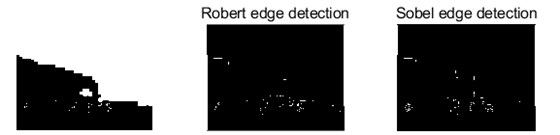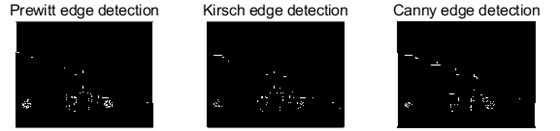Table 1Evaluation summary of five detection operators

 The serial number The name of the operator Order number Noise sensitivity Positioning accuracy Signal to noise ratio 1 Sobel The first order Higher Low Low 2 Roberts The first order Higher Higher Higher 3 Canny The first order High Higher Higher 4 Prewitt The first order Higher Low High 5 Kirsch The first order High Low Low

In view of the above experimental analysis, this paper finally chooses Canny operator for image edge detection.

## 3. Detection and recognition of traffic signal lights

The detection of traffic signal light is actually the process of collecting the required images from the camera. The color of the traffic signal light can be recognized by algorithm recognition of the detected image .

### 3.1. Traffic light detection

Traffic light recognition system is a multi - sensor fusion system. In order to identify the traffic signal lights, the first thing to do is to detect the required image through a reasonable detection device, so as to carry out the subsequent recognition work .

The vision sensor is a sensor that carries on the image processing to the image taken by the camera, detects the target, and outputs the data and the judgment result. The application of vision sensors in intelligent networked vehicles or driverless vehicles is in the form of cameras (machines), which are equipped with advanced artificial intelligence algorithms to facilitate target detection and image processing.

According to the different lens and layout, the vision sensor is mainly divided into the following four kinds: monocular camera, binocular camera, three-ocular camera and round-looking camera. Monocular camera is characterized by its low cost, the ability to identify specific categories of obstacles, high algorithm maturity, accurate identification; The binocular camera needs two cameras to have high synchronization rate and sampling rate. The technical difficulty lies in binocular target positioning. Compared with the former two, the three-camera camera has a larger perception range, but it needs three cameras, which means a large workload. The camera is mainly used for autonomous parking and obstacle detection. Combined with the above analysis, this paper selects a camera.

### 3.2. Traffic light recognition

For the identification of traffic signal lights, the functions should be: image acquisition of the spatial distribution of traffic signal lights, and then preprocessing of the collected images, using Canny operator for edge detection, and finally, according to the method of extracting pixel points to identify the color of traffic signal lights . The advantage of this method is that the identification process is simple and the identification speed is fast. The designed algorithm process is shown in Fig. 2.

Fig. 2Process diagram of the algorithm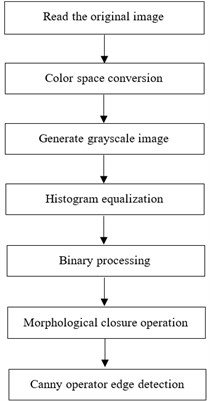### 3.2.1. Color space conversion

As shown in Fig. 3, the HSV color space represents the points in the RBG color space in an inverted cone. The color space uses three channels to represent color: Hue, Saturation and Value. HSV color space uses the Angle to measure the color, 0° represents red, counterclockwise calculation, 60°, 120°, 180°, 240°, 300° represents yellow, green, cyan, blue and magenta respectively. Saturation refers to the purity of the color, and the value of this channel is defined by percentages in the range [0, 1].

Fig. 3HSV color space model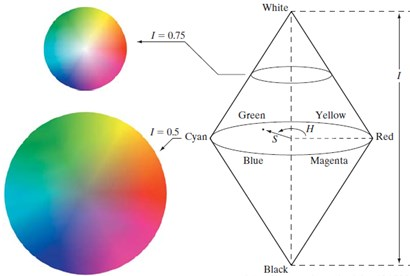As can be seen from Fig. 3, the higher the saturation value, the brighter the color will be; the lower the saturation value, the darker the color will be. Lightness indicates the brightness of the color. For the light source, lightness is related to the brightness of the light emitting body. For objects, lightness is related to the reflectivity of the object .

Compared with the RGB color space used by computer and TV display, HSV color space is not suitable for the display system, but more in line with the visual characteristics of human eyes. Therefore, color will be converted from the RGB space domain to the HSV space domain for processing. The processing graph after the original image is converted to the HSV color space is shown in Fig. 4.

Fig. 4HSV color space conversion images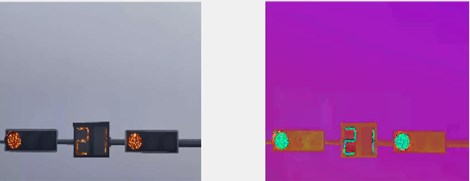As can be seen from Fig. 4, the image converted into HSV space can more intuitively express the light and shade, tone and brightness of color, so as to facilitate the contrast between colors.

### 3.2.2. Gray image

The process of transforming color image into grayscale image is called image grayscale processing. The color of each pixel in a color image is determined by three components, R, G and B. Each component is divided into 256 levels from 0 to 255, and there are 256 values that can be taken. In this way, a pixel can have a color range of more than 16 million. However, grayscale image is a special color image with the same components of R, G and B, and the variation range of a pixel is 256. Therefore, in digital image processing, images of various formats are generally converted into grayscale images in order to reduce the amount of computation of subsequent images. The description of grayscale image, like the color image, still reflects the distribution and characteristics of the overall and local chromaticity and brightness levels of the whole image.

The grayscale image generated after the color space conversion is shown in Fig. 5.

Fig. 5Gray image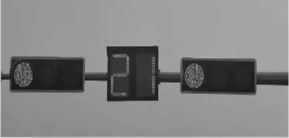As can be seen from Fig. 5, after gray processing, the image can be simplified and the computing speed can be improved.

### 3.2.3. Histogram equalization

According to the existing gray image histogram equalization. The basic idea of histogram equalization is to transform the histogram of the original image into the form of uniform distribution, so as to increase the dynamic range of pixel gray value so as to achieve the effect of enhancing the overall contrast of the image.

The histogram equalized image is obtained, as shown in Fig. 6 and Fig. 7.

According to Fig. 6 and Fig. 7 shows, compared to before the gray image, histogram equalization processing, less the original pixel gray level will be assigned to other grayscale pixels is relatively concentrated, after processing grayscale range, contrast, sharpness, can effectively enhance image display effect, improve the accuracy of recognition.

Fig. 6Histogram equalization image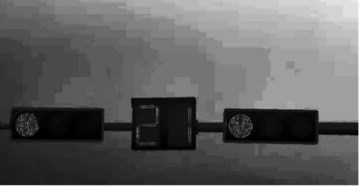Fig. 7Histogram comparison before and after equalization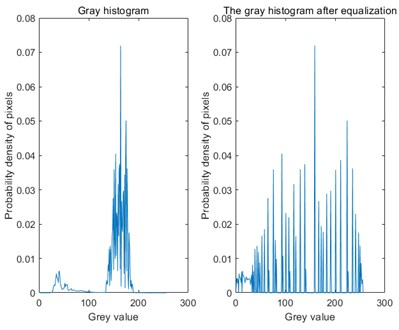### 3.2.4. Threshold image

An image includes the target object, the background and the noise. In order to directly extract the target object from the digital image, the most commonly used method is to set a threshold $T$, and use $T$ to divide the image data into two parts: the pixel group greater than $T$ and the pixel group less than $T$. This is the most special method to study the gray transformation, called image binarization . The image after binarization is shown in Fig. 8.

Fig. 8Threshold image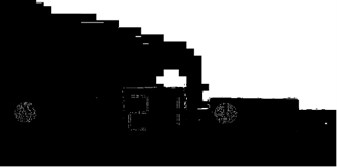As can be seen from Fig. 8, the gray value of the binarization image has no intermediate transition image, and its advantage for the identification of traffic signal lights is that it takes up less space and excludes other interference factors.

### 3.2.5. Morphological processing

In this paper, morphological closure operation was used to fill the image, smoothing its boundary while basically keeping its area unchanged. After the morphological closure operation, the image appears more full, filling the gap, improving the accuracy of recognition, and making the extracted points more accurate . The results after morphological closing operation are shown in Fig. 9.

Fig. 9Morphological closure results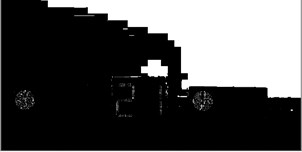### 3.3. Application of Canny operator in traffic signal light recognition

Firstly, the parameters in the image processed by Canny operator are selected as the basis of the analysis.

The image of traffic signal lamp has the characteristics of isolated noise and continuous noise, among which the isolated noise is caused by the imaging sensor, photo particles, light imbalance, error in the process of image transmission and other factors. Therefore, Gaussian smoothing filter is applied to the original image to smooth the image and filter out the noise. The gradient intensity and direction of each pixel point are then calculated separately . Fig. 10 shows the gradient image calculated using the Canny operator, and the gradient Angle ranges from –180° to 180°.

Non-extremal suppression technology is used to eliminate the influence of stray edges, and double threshold detection is used to determine the real and potential edges. Finally, edge detection is completed by suppressing isolated weak edges, and the Canny edge detection processing image is obtained. See Fig. 11.

Fig. 10Gradient image using Canny operator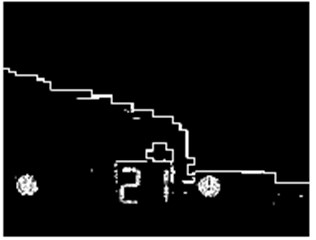Fig. 11Canny operator after detection resultsBy Canny operator image processing results show that the Canny operator can more effectively reduce the influence of noise, and the detected edge contour is obvious, clear, good continuity, while accurately detect the image edge and suppresses the false edge well, is conducive to further analysis and processing in traffic light image, which reflects the advantages of the Canny algorithm chosen in this paper.

## 4. The MATLAB simulation

In this paper, the identification scheme specifically for traffic lights is simulated in MATLAB software. After identifying a large number of pictures taken, the result can identify the pixel points of red, green and yellow. After that, the number of red, yellow and green pixel points is output through the histogram drawn, among which the color with the largest number is the color of the recognized traffic signal lamp, and the color recognition result of the traffic signal lamp is obtained.

The algorithm was tested on a computer with a CPU frequency of 2.6 GHz and a memory of 8G. The image size collected was 1440×1080, and the running environment was MATLAB 2019B. The images collected include different time periods (day and night) and different disturbance environments (the complexity of disturbance environments such as vehicles, trees and streetlights).

1) Accuracy.

Detection rate refers to the proportion of accurate color judgment of traffic signal lights, and detection rate $T$ is defined as follows:

15
$T=\frac{TD}{SUM},$

where: $TD$ – detects the correct number of images; $SUM$ – the total number of images.

The experimental result is 98 %, indicating that the detection rate of the all-day real-time traffic signal light recognition algorithm is high, the recognition rate reaches more than 90 %, and the recognition effect is good .

2) Real-time comparison.

The five operators are compared in terms of running time and recognition time, and the results are shown in Table 2.

Table 2Time comparison of the five operators

 Sobel Roberts Canny Prewitt Kirsch Running time (s) 0.036585 0.052018 0.059339 0.035864 0.041885 Time of recognition (s) 0.37560 0.44830 0.62350 0.31790 0.40200

As can be seen from Table 2, the running time of Canny operator is longer than that of other operators, averaging 0.01775 s longer than that of other operators. In addition, the time required for the overall recognition process is longer, averaging 0.2375 s longer than that of other operators. However, the image edge processed by this algorithm is less affected by noise, and the running time is not much longer than other operators, but the accuracy is greatly improved compared with other operators, and it is suitable for the identification of traffic lights in urban environment.

3) Algorithm stability.

Traffic light recognition algorithm based on Canny operator can adapt to images in any time period throughout the day and is suitable for urban environment. Table 3 and Fig. 12 are the results of a correctly identified traffic signal light in the experimental test.

Table 3The number of red, yellow and green pixel colors

 Color Number of pixels Red 211 Yellow 37 Green 4

As can be seen from the above experimental results, the number of red dots identified is 211, the number of green dots is 4, and the number of yellow dots is 37, so the color of the traffic signal light is red, and the identification result is correct and reliable [17-18].

The recognition effect of traffic signal lamp based on Canny operator is better. Compared with the methods studied before, it can ensure the stability of the program and can quickly identify the signal lights with high accuracy.

Fig. 12Identify successful traffic light results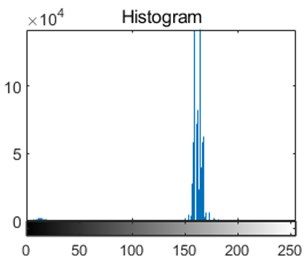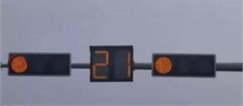## 5. Conclusions

This paper mainly studies the detection and recognition of traffic signal lights based on Canny edge detection operator, introduces five kinds of classical edge detection operators, and finally chooses Canny operator for edge detection. In this paper, the research content is divided into two stages, including detection stage and identification stage, through the vision sensor for the traffic signal lamp image acquisition, the image from RGB space into HSV space, the image grayscale, histogram equalization, binarization processing, morphology closed operation, Canny operator edge detection, Finally, by extracting the number of pixel points, the color of the traffic signal light can be accurately identified. The feasibility and accuracy of the proposed algorithm are verified by the simulation of a number of actual intersection traffic lights taken on MATLAB platform. After comparing with the schemes of previous scholars, the advantages of this paper are that the whole process is relatively simple and the identification accuracy is high. The identification of traffic signal lights can be carried out and the success rate of the identification of traffic signal lights is high.